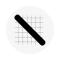# Slope Calculator

Install latest/stable of Slope Calculator

Ubuntu 16.04 or later?

Make sure snap support is enabled in your Desktop store.

Install using the command line

``sudo snap install slopecalculator``

Don't have snapd? Get set up for snaps.

Channel Version Published

#### Get accurate results for finding slope

What is Slope Calculator?

Slope Calculator is a powerful macOS tool that allows you to calculate the slope of a line, given two points on the line. With Slope Calculator, you can quickly and easily find the slope of a line, even if you don't have a calculator handy.

How to use Slope Calculator to find slope:

Finding the slope of a line is easy with Slope Calculator. Here's how to do it:

Step 1: Select the method.

The Slope finder offers two different methods for calculating the slope of a line, first select the method from the drop-down menu.

Step 2: Input.

If you have selected the “two points” method, then enter points X1, X2, Y1, and Y2 in the designated input boxes. If you have selected the “Line Equation” method, then simply enter the corresponding values of X, Y and C.

Step 3: Calculate.

Press “Enter” or click on the “Calculate” button to calculate the result. If you want to start over, click on the “reset” button or hit the “Start over” button.

Benefits of Using Slope Calculator:

There are many benefits to using Slope Calculator, including:

Saves time:

With Slope Calculator, you can solve slope problems quickly and easily, saving you time and effort.

Accuracy:

Slope Calculator is designed to be accurate, so you can trust the results you get.

User-friendly:

Slope Calculator is user-friendly and easy to use, even if you're not a math expert.

Versatility:

Slope Calculator can be used to solve a wide range of slope-related problems, including finding slope, calculating slope using the slope formula, and solving slope-intercept form equations.

#### Details for Slope Calculator

• unset

##### Last updated
• 27 April 2023